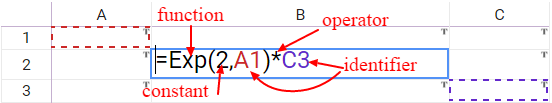# Overview of qalcwise formulas

Qalcwise formula language allows to perform complex calculations and implementing not trivial business logic in applications.

There are many places in qalcwise where formulas can be used to calculate value, spreadsheet cells, section options, workflow parameters .etc, complete list can be found in this article: Where can I use formulas?

Formulas in qalcwise start with = followed by numbers, constants, operators and built in functions.

For example, subtracting 4 from 12, and then divide it by 2 is:

=(12-4)/2

More examples:

=10+A1+15 - adds 10 value in cell A1 and 15

=A1+Section2.A5 - adds value in cell A1 and value in cell A5 in Section 2

=CONCAT(‘prefix’, B3) - concatenates ‘prefix’ with value in cell B3

=NOW() - returns current time

=IF(A1>A2, A3, A1<A2, A4, A5) - if value in A1 is greater that value in A2 returns value from A3, else if value in A1 and less than value in A2 return A4, else return A5

Strong Typing

Formulas in qalcwise always return value of a specific type, this can be Text, Number, Boolean (true or false) or Date, a place where formula is written determines a resulting type, for example, a cell number requires formula that returns a number.

Elements of formulas

Formulas in qalcwise consist of the following elements: operators, functions, constants, identifiers (addresses)• Exp is function rises 2 to power of A1
• 2 is constant
• A1 and C3 are identifiers - cell addresses in this instance
• * is multiplier operator

Operators

Operators define operation that will be calculated between parts of formula, qalcwise follows mathematical rules of operators precedence, a user can change this precedence by using parentheses. Example: 4 + 2×3 = 4 + (2×3) = 4 + 6 = 10.

All operators by type:

• Arithmetical operators:
• - subtract 4-4 or negate -4
• * multiply 4*4
• / divide 4/4
• % modulo - returns the rest from dividing 25%2
• Comparison operators:
• = equals 4=4
• > greater 4>3
• < less 3<4
• != not equal 3!=4
• >= greater or equal 4>=4
• <= less or equal 4<=4
• Logical operator
• && and and
• || or or

Constants

Constants are static values, for example: 2, 123, ‘text value in single quotes’, true, false

Functions

Qalcwise offers a rich library of functions, starting with math functions, text functions, dates functions and adding on functions related to workflow process.

Functions can be nested in each other, function parameter can be provided as another function result value, for example:

=exp(2, (exp(2, 2))

Function parameters are separated by a colon character.

Identifiers

Identifiers are last type of formula components, identifiers are:

• Cell address - A1, A2, inside one section
• Section + Cell Address - A1, Section.A2 - identifies cell in any context
• Column address - A, B, C, scalar value in the context of one section (used inside a section it relates to)
• Section + Column address, A, Section1.B - an array of values in a table section column
• Range of cells - A1:A8, an array of values in a range of cells, in context of one section
• Section + range of cells, B6:B18, an array of values in a range of cells in any context
• Input parameter name: Id, User as is defined in app definition
• Data object name: List Name, Data Table Name, App Name: for example: SYSUSER, PRODUCT, LEAVES_RESUEST
• Data object column/alias: List column, Data Table Column, App Alias - can be used in formulas inside bounded section, returns value from bounded object column/alias

Array values

Some formulas and some identifiers are of array type, the array type is not a valid type for a cell content, but can be a valid type for Workflow ActionStep parameter, for example, SendMail Action step accepts array for To, Cc and Bcc parameters.

Examples of functions that work with the array type parameters:

=Array(1,3,5) - creates array value

=Nth(A1:A6, 2) - return nth (in this instance second) value from array

=First(Section1.B) - returns first value from column B in section 1

=Sum(Section1.D) - sums section 1 column D values